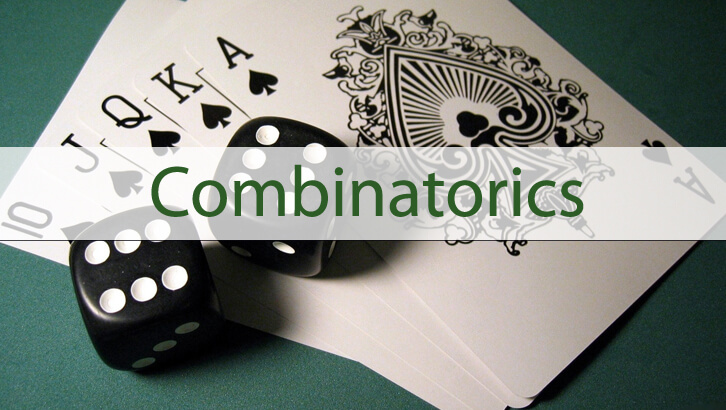# Solutions to Number Theory and Counting questions

Given below are the solutions to these Number theory questions.

1. From the digits 2,3,4,5,6 and 7, how many 5-digit numbers can be formed that have distinct digits and are multiples of 12?

Any multiple of 12 should be a multiple of 4 and 3. First, let us look at the constraint for a number being a multiple of 3. Sum of the digits should be a multiple of 3. Sum of all numbers from 2 to 7 is 27. So, if we have to drop a digit and still retain a multiple of 3, we should drop either 3 or 6.

So, the possible 5 digits are 2, 4, 5, 6, 7 or 2, 3, 4, 5, 7.

When the digits are 2, 4, 5, 6, 7. the last two digits possible for the number to be a multiple of 4 are 24, 64, 52, 72, 56, 76. For each of these combinations, there are 6 different numbers possible. So, with this set of 5 digits we can have 36 different numbers

When the digits are 2, 3, 4, 5, 7. the last two digits possible for the number to be a multiple of 4 are 32, 52, 72, 24. For each of these combinations, there are 6 different numbers possible. So, with this set of 5 digits we can have 24 different numbers

Overall, there are 60 different 5-digit numbers possible

2. All numbers from 1 to 200 (in decimal system) are written in base 6 and base 7 systems. How many of the numbers will have a non-zero units digit in both base 6 and base 7 notations?

If a number written in base 6 ends with a zero, it should be a multiple of 6. In other words, the question wants us to find all numbers from 1 to 200 that are not multiples of 6 or 7. There are 33 multiples of 6 less than 201. There are 28 multiples of 7 less than 201. There are 4 multiples of 6 & 7 (or multiple of 42) from 1 to 200.

So, total multiples of 6 or 7 less than 201 = 33 + 28 – 4 = 57. Number of numbers with non-zero units digit = 200-57 = 143.

3. All numbers from 1 to 150 (in decimal system) are written in base 6 notation. How many of these will not contain any zero?

Any multiple of 6 will end in a zero. There are 25 such numbers. Beyond this, we can have zero as the middle digit of a 3-digit number. This will be the case for numbers from 37-41, 73-77, 109-113 and 145-149. There are 20 such numbers. Overall, there are 45 numbers that have a zero in them.

4. How many factors of 1080 are perfect squares?

1080 = 2^3 * 3^3 * 5. For any perfect square, all the powers of the primes have to be even numbers. So, if the factor is of the form 2^a * 3^b * 5^c. The values ‘a’ can take are 0 and 2, b can take are 0 and 2, and c can take the value 0. Totally there are 4 possibilities. 1, 4, 9, and 36.